Calculators and Useful Tables

# Calculators and Useful Tables

1 , 2 , 3 > >>

SWR table and Calculator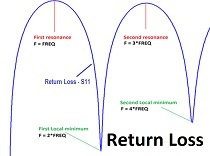The Impedance table of the Inner Homogeneous Stripline vs. FR4 Thickness (H), FR4 Dielectric Constant (Ԑr) and Stripline Width (W) - 50 Ohms.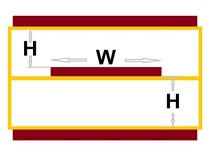The modern PCB layout quite often needs accurately calculate an impedance of inner conductors, (basically means 50 Ohms matched transmissions lines). As we know it should be done before when the layer stack-up will be approved.

Unfortunately, here a headache begins, because needs to find a compromise between line width and thickness, FR4 thickness and dielectric constant. Also, needs to find a compromise between the PCB house’s capabilities and the desired PCB's layer stack-up.

This table should be helping you in this case.

Determination of the relative permittivity (Ԑeff) of microstrip transition lines.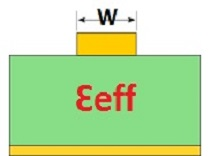Design RF/microwave or High Speed Signal PCB, often needs to determinate the relative permittivity (Dielectric Constant Ԑeff) of microstrip transition lines (PCB’s top and bottom). For this useful the left part of this calc.

The right side of this calc can be used for determination of the Dielectric Constant (Ԑr) of FR4 material. For this needs to have a measuring line on the board or on the panel.

The Quarter Wave Length Calculator. Introducing and calculation.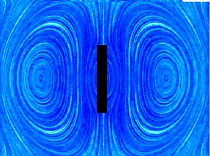Resistors in practice. Three interesting calculators.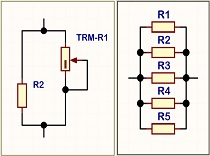Two of them (Resistor-1 and Resistor-2) can help determine the right value of a POT in a fine-tuning rheostat circuit with an additional paralleled shunt resistor (resistors).

Also, it allows define accurate fixed deviation (+/-) of the resultant resistance in percent.

Even more - you can see in which position will be the central pin of the POT depending on the resultant resistance.

An example: need the rheostat 5k with deviation +/- 10% (4.5k - 5.5k). The 100k POT was chosen.

The “Resistor-1” calc shows:

• Total resistance MAX would be 5.500k;
• R additional (R2) should be 5.82k;
• The POT would work from 20 to 100%;
• The POT has middle position 50% close to middle total (resultant) resistance = 5.213k;

Third Resistor-3 calc helps calculate resistors in parallel – up to five.

The potentiometer design. Three calculators.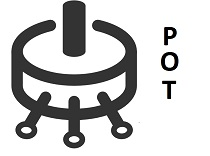• Potentiometer 64 linear calc allows calculate any resistors potentiometer up to 64 stages;
• Potentiometer -32dB calc helps calculate the logarithmic potentiometer 0 / -32dB with 1dB step;
The dB calculator: dBu, dBm, Nepers.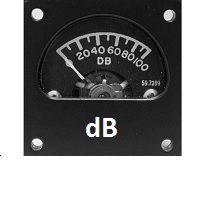Features:

• VA ratio to dB, dBu and Nepers conversion;
• dB, dBu and Nepers to VA ratio conversion;
• dBu and V ratio to V conversion (600 Ohms / 1mW);
• dB to % (ratio) conversion; useful for THD;
• Power ratio to dBm conversation;
• dBm to Power ratio conversation;
• dBm and Power ratio to Watts conversion - (50 Ohms / 1mW);

Also, refer to three tables:

1 , 2 , 3 > >>